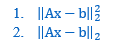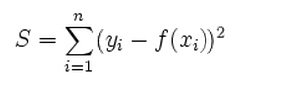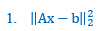# L2 Norm Understanding

When I run the following in CVX

cvx_begin
variable x(n)
minimize( norm(A*x-b,2) )
subject to
l <= x <= u
cvx_end

does it solved 1 or 2 ?My understanding is it solve number 2.

And is it equivalent to solving S or squareRoot of S where;I have read somewhere that L2 norm is square root of S, is that correct?

is Number 2 equivalent to S or Square-root of S ? i.e. is L2 norm equivalent to solving S or Square-root of S.

Number 2 is square root of S

If I want to implementis the follwoing an accurate implementation ?

cvx_begin
variable x(n)
minimize power(norm(A*x-b,2),2 )
subject to
l <= x <= u
cvx_end

That will produce an error.

Use
`minimize(square_pos(norm(A*x-b,2)))`
or
`minimize(sum_square(A*x-b))`

There are also other ways of doing it.

I will recommend doing

minimize(norm(A*x-b,2))

over

minimize(sum_square(A*x-b))

The first one has better numerical properties IMO.

Can you explain what kind of numerical properties are you taking about? Also, since am solving a constraint least square problem so solving `minimize(square_pos(norm(A*x-b,2)))` isn’t more appropriate?

Squaring the norm is basically squaring (hence increasing) the condition number, which essentially takes away digits of accuracy in the solution process, hence making it less numerically stable.

If the norm (or its square) is the only term in the objective function, then the argmin (if exactly computed) will be the same for the squared or not versions. That is true whether or not there are any constraints. However, if there is an additional term in the objective function, then squaring or not the norm term can change the argmin.

I believe this is what @Erling was getting at. I suppose I should feel guilty for not pointing this out (but thank Erling for doing so), just as I should feel guilty for thinking about, but being too lazy to point out earlier in the day of my post in this thread, that a QCQP would be better reformulated as an SOCP for the same reason as discussed in this thread. But I post more than enough, so I don’t feel guilty.